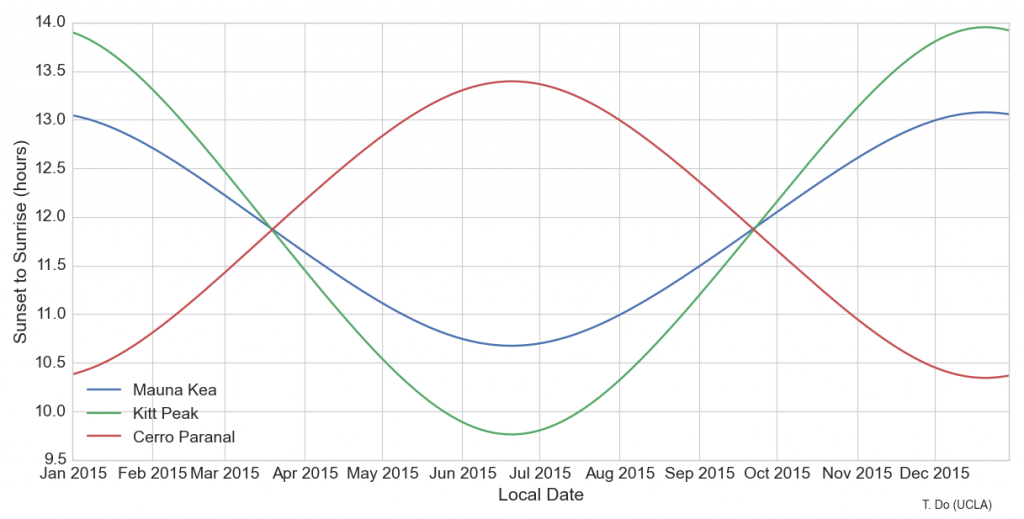# Hours between sunrise and sunset at different observatories with python

By T. DoThe plot above shows the number of hours between sunrise and sunset at three different locations for observatories around the world. Mauna Kea, Hawaii is home to a number of telescopes including the Keck Telescopes, Gemini, and Subaru. Kitt Peak is a mountain outside of Tuscon and includes the Mayall Telescope and WIYN telescope. Cerro Paranal is a mountain in Chile with the four VLTs. The variation in night time depends on the latitude of the location on Earth. Because Mauna Kea in Hawaii is closer to the equator, there is less variation in the number of night time hours.

To create this plot, I used the python package Astral and datetime. Below I will discuss in more detail how to use these packages.

Astral is a python package for calculating sun rise and sun set at different locations. It includes an internal list of some cities on Earth, but it also includes a very handy interface for Google Geocode so that you can use it for less common locations, as in our case of observatories. The Astral documentation has information about how to get started, so I would just like to add the part about using the Google Geocoder. Here is an example of using Astral with the package’s Google Geocoder:

```from astral import Astral, GoogleGeocoder

location = a.geocoder["Mauna Kea"]
print(location)```

You should get the following output:

`Mauna Kea/Hawaii, USA, tz=Pacific/Honolulu, lat=19.82, lon=-155.47`

To determine the time of sunrise or sunset, as well as twilight, use the `sun()` method. You can specify the definition of twilight with the `solar_depression` attribute. It accepts `civil, nautical, or astronomical`, corresponding to 6, 12, and 18 degree twilight.

```a.solar_depression = 'nautical' # 12 deg twilight for dusk and dawn calculations
s1 = loc.sun(datetime.date(2015,5,5),local=True)```

`s1` should be a dictionary containing the following `datetime` objects:

```{'dawn': datetime.datetime(2015, 5, 5, 5, 27, 11, tzinfo=<DstTzInfo 'Pacific/Honolulu' HST-1 day, 14:00:00 STD>),
'dusk': datetime.datetime(2015, 5, 5, 19, 9, 53, tzinfo=<DstTzInfo 'Pacific/Honolulu' HST-1 day, 14:00:00 STD>),
'noon': datetime.datetime(2015, 5, 5, 12, 18, 33, tzinfo=<DstTzInfo 'Pacific/Honolulu' HST-1 day, 14:00:00 STD>),
'sunrise': datetime.datetime(2015, 5, 5, 5, 50, 24, tzinfo=<DstTzInfo 'Pacific/Honolulu' HST-1 day, 14:00:00 STD>),
'sunset': datetime.datetime(2015, 5, 5, 18, 46, 40, tzinfo=<DstTzInfo 'Pacific/Honolulu' HST-1 day, 14:00:00 STD>)}```

As an aside, the python datetime package is very useful for representing time and doing basic arithmetic with time. The arithmetic works by returning a `timedelta` object when ever two dates are either added or subtracted. This `timedelta` object has a method, `timedelta.total_seconds()` that converts this time interval into seconds.

For reference, below is the python code used to generate the plot above. You can also use it to generate the time interval between twilight (probably more useful for astronomers) by plotting the `dusk_dawn` list instead. The code uses the seaborn for fancier plots, but those parts can be commented out.

```import numpy as np
import pylab as plt
import seaborn as sns
from astral import Astral, AstralError
import datetime

def plot_night_duration():

a.solar_depression = 'nautical' # 12 deg twilight for dusk and dawn calculations
locations = ['Mauna Kea', 'Kitt Peak', 'Cerro Paranal']
startdate = datetime.date(2015,1,1)
enddate = datetime.date(2015,12,31)
timeinterval = datetime.timedelta(1)  # how finely to sample the time
for i in xrange(len(locations)):
loc = a.geocoder[locations[i]]

print(loc)
currentdate = startdate
sunset_sunrise = []
dusk_dawn = []
dates = []
while (enddate - currentdate).total_seconds() > 0:
try:
s1 = loc.sun(currentdate,local=True)
s2 = loc.sun(currentdate+datetime.timedelta(1),local=True)
sunset_sunrise.append((s2['sunrise']-s1['sunset']).total_seconds()/3600.0)
dusk_dawn.append((s2['dawn']-s1['dusk']).total_seconds()/3600.0)
except AstralError:
# there is a problem if the sun doesn't rise or set on the same day
sunset_sunrise.append(24.0)
dusk_dawn.append(24.0)

dates.append(currentdate)
currentdate = currentdate+timeinterval

if i == 0:
plt.figure(1,figsize=(12,6))
plt.clf()

sns.set_style('whitegrid')
sns.set_context('paper',font_scale=1.75, rc={"lines.linewidth": 1.75})

plt.plot(dates,sunset_sunrise,label=locations[i])
plt.xlabel('Local Date')
plt.ylabel('Sunset to Sunrise (hours)')
plt.legend(loc=3)
plt.annotate('T. Do (UCLA)',(.9,0.02),xycoords='figure fraction',fontsize=10)```